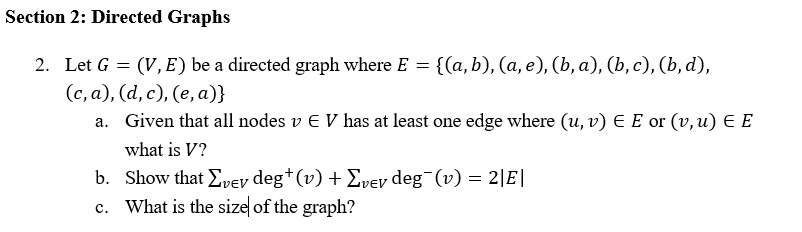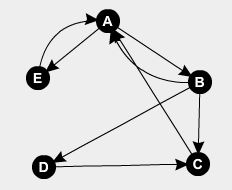# Question & Answer: Directed Graphs Let G = (V, E) be a directed graph where E = {(a, b), (a, e), (b, a), (b, c), (b, d), (c, a) (d, c), (e, a)} a. Given that all n…..Directed Graphs Let G = (V, E) be a directed graph where E = {(a, b), (a, e), (b, a), (b, c), (b, d), (c, a) (d, c), (e, a)} a. Given that all nodes v elementof V has at least one edge where (u, v) elementof E or (v, u) elementof E what is V? b. Show that sigma_v elementof v deg^+ (v) + Sigma_v elementof v deg^-(v) =2 |E| c. What is the size of the graph?

Don't use plagiarized sources. Get Your Custom Essay on
Question & Answer: Directed Graphs Let G = (V, E) be a directed graph where E = {(a, b), (a, e), (b, a), (b, c), (b, d), (c, a) (d, c), (e, a)} a. Given that all n…..
GET AN ESSAY WRITTEN FOR YOU FROM AS LOW AS \$13/PAGE

Given edges of directed graph G = (V, E) = {(a,b),(a,e),(b,a),(b,c),(b,d),(c,a),(d,c),(e,a)}

So the graph will be ——>a. Given that all nodes v  V has at least one edge where (u,v)  E or (v, u)  E then V are —-> (a, b, c, d, e)

because we have (a,b) & (b,a) and (a,e) & (e,a)

b. For given graph indegree for vertex (a,b,c,d,e) = (3,1,2,1,1)

and outdegree for vertex (a,b,c,d,e) = (2,3,1,1,1)

Total of indegree and outdegree = (3+1+2+1+1)+(2+3+1+1+1) = 8+8 = 16

Total edges = 8

2 * E = 2 * 8 = 16

So total number of indegree + total number of outdegree = 2|E|

c. The size of a graph is |E| = 8